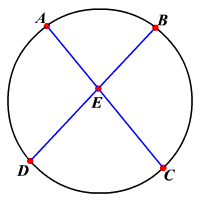# Intersecting Chords Theorem

If two chords intersect in a circle , then the products of the measures of the segments of the chords are equal.In the circle, the two chords $\stackrel{¯}{AC}$ and $\stackrel{¯}{BD}$ intersect at point $E$ .

So, $AE\cdot EC=DE\cdot EB$ .

Example :

In the circle shown, if $AE=7,EC=12$ and $BE=8$ , then find the length of $DE$ .

Substitute.

$\begin{array}{l}AE\cdot EC=DE\cdot EB\\ \text{\hspace{0.17em}}\text{\hspace{0.17em}}\text{\hspace{0.17em}}\text{\hspace{0.17em}}\text{\hspace{0.17em}}\text{\hspace{0.17em}}\text{\hspace{0.17em}}7\cdot 12=DE\cdot 8\\ \text{\hspace{0.17em}}\text{\hspace{0.17em}}\text{\hspace{0.17em}}\text{\hspace{0.17em}}\text{\hspace{0.17em}}\text{\hspace{0.17em}}\frac{7\text{\hspace{0.17em}}\cdot \text{\hspace{0.17em}}12}{8}=DE\\ \text{\hspace{0.17em}}\text{\hspace{0.17em}}\text{\hspace{0.17em}}\text{\hspace{0.17em}}\text{\hspace{0.17em}}\text{\hspace{0.17em}}\text{\hspace{0.17em}}\text{\hspace{0.17em}}10.5=DE\end{array}$

Therefore, $DE=10.5$ units.